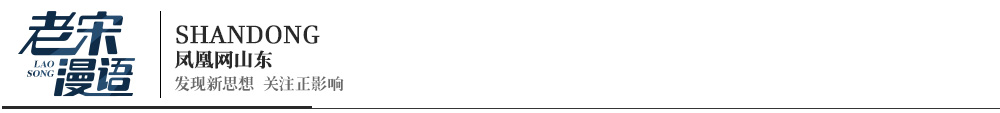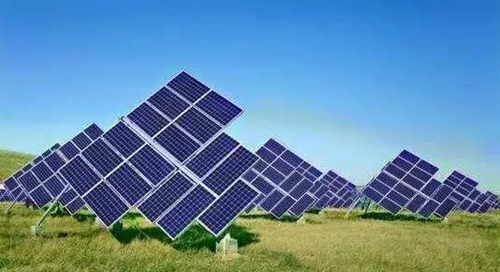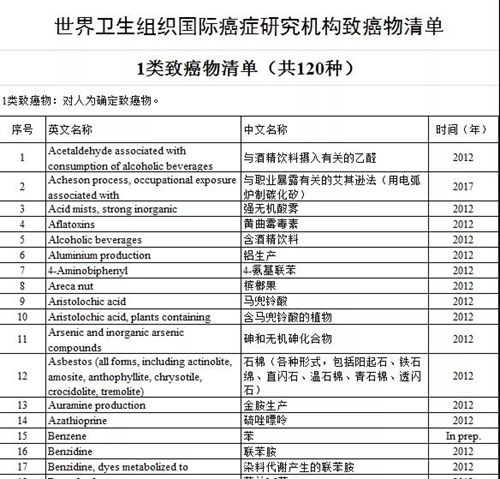# 辞猪迎鼠话常识

2020.1.21 第5期 作者：宋传杰

P（红色） = V / R * V = 110/9 *110 = 1344

P（黑色） = V / R * V = 220/25*220 = 1936

P（红色） = V / R * V = 220/9 * 220 = 5377

P（黑色） = V / R * V = 110/25 * 110 = 0484

● 红色应该接市电、黑色应该接光伏，现在接反了。

● 我测量了，直流功率是1560W，交流功率是3000W。

● 电热器的阻抗冷态和热态不一样。

P（红色） = V / R * V = 220/9 *220 = 5377

P（黑色） = V / R * V = 110/25*110 = 0484

220V 接红色（9Ω），变成了3000W；

110V接25Ω，就应该是约270W（图片来自网络）（图片来自网络）